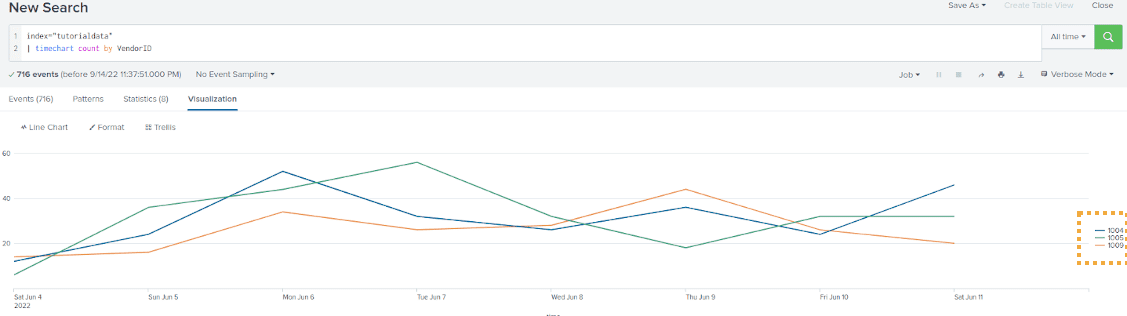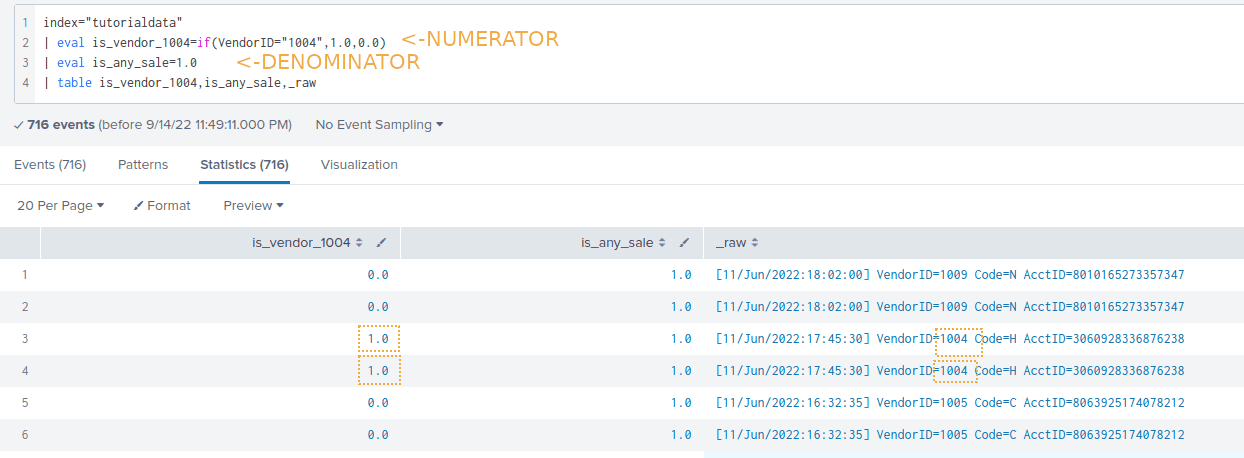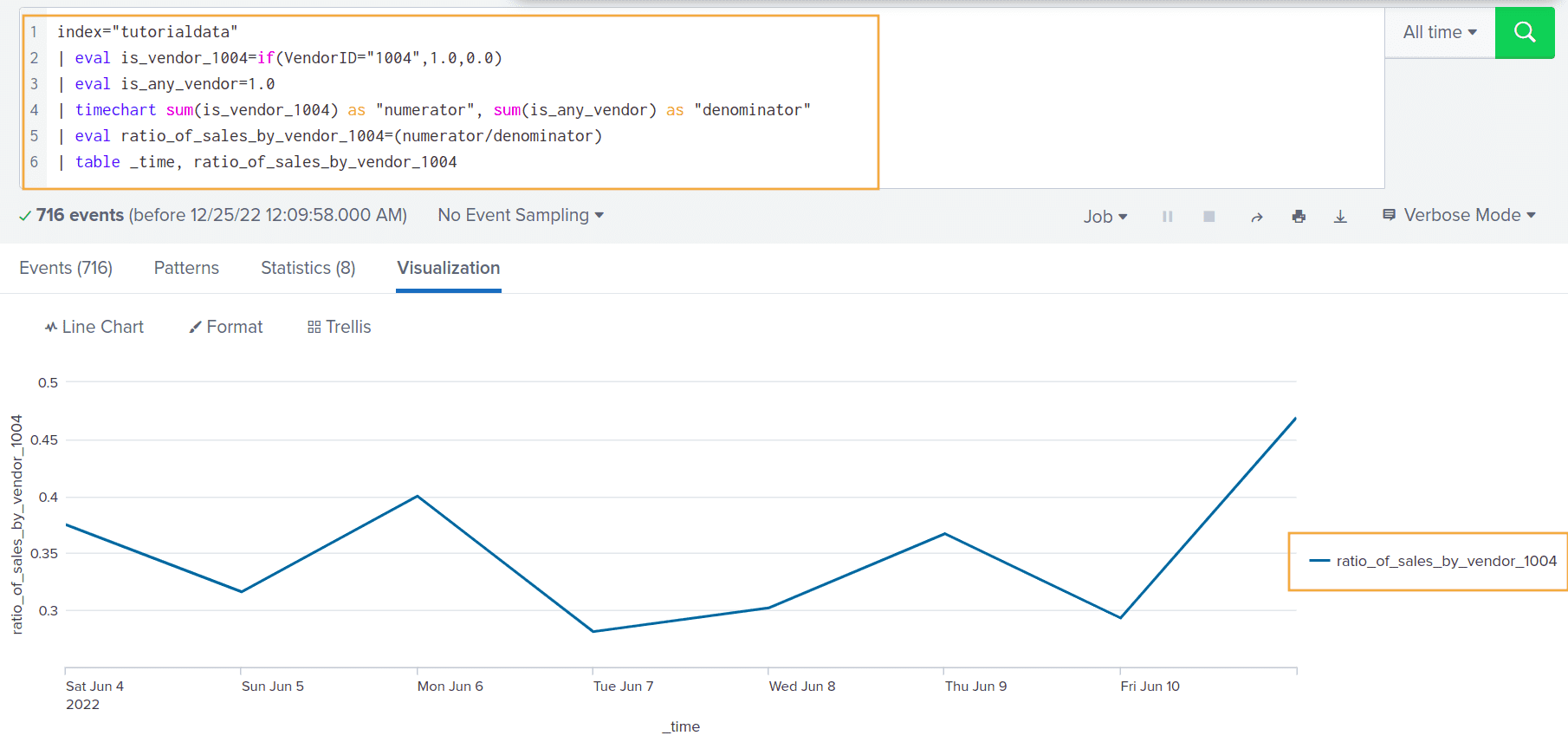Splunk Examples: Calculating Ratios Between Events

# Splunk Examples: Calculating Ratios Between Events

Last updated:

All examples use the tutorial data from Splunk running on a local Splunk version

## Calculate ratio between events

Use this template, replacing the values within < and > with your own values

index="tutorialdata"
| eval numerator=<IF_CLAUSE_REPRESENTING_THE_NUMERATOR>
| eval denominator=<IF_CLAUSE_REPRESENTING_THE_DENOMINATOR>
| timechart sum(numerator) as "numerator", sum(denominator) as "denominator"
| eval ratio=(numerator/denominator)
| table _time, ratio


Calculating ratios and percentages between different events is useful for showing:

• timeout rates
• failure rates
• ratios between two quantities

For this example, suppose you have this log for the number of sales by each VendorID, per unit time):

index="tutorialdata"
| timechart count by VendorIDThree events taking place

Ỳou now want to plot the ratio between VendorID 1004 and the total, to see what fraction of the total sales was made by 1004:Create one variable for the numerator and another one for the denominator like this

Example: Plot the ratio of sales made by Vendor 1004 over time:

index="tutorialdata"
| eval is_vendor_1004=if(VendorID="1004",1.0,0.0)
| eval is_any_vendor=1.0
| timechart sum(is_vendor_1004) as "numerator", sum(is_any_vendor) as "denominator"
| eval ratio_of_sales_by_vendor_1004=(numerator/denominator)
| table _time, ratio_of_sales_by_vendor_1004The rate of sales made by Vendor 1004 ranges
from 0.281 to 0.469, depending on the day!

(Note that we call timechart and then table at the end)